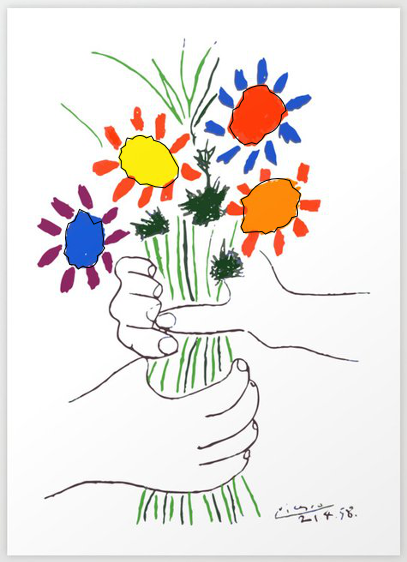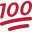# B4J Tutorial[B4X]Turning Miscellaneous Image Shapes into Buttons

This is just a bit of fun, inspired by a recent question about the shape of buttons.
Given an image, how hard is it to make a specific area in the picture sensitive to clicks.

It turns out "easy", if the areas have fairly uniform color(s).
The project (about 100 lines of code) uses these techniques:

During the definition phase...
1. Create 2 layers (panels) one for the image and one for the mouse click sensor
2. In response to a click, sample a small rectangle of color using BitmapCreator
3. Use a radial search to detect boundaries (search centered on event point, around the clock in 10 degree steps)
4. Using a bcpath object, connect boundaries and fill BitmapCreator object with encoded color
5. Put the area ID# in the shape's color channels (1, 2, 3, 4, etc.)

During the testing phase...
1. grab the color of a clicked point form the BitmapCreator object to get the shape ID#
2. show a message about the selected shape

I tried to attach the project as .zip, but it was too large. So just copy the following code in a standard template.
Add the attached .png file as an asset using the Files Manager tab.

I have tested it on B4j, but it should work on other platforms with an adjustment for the mouse event.

When testing, in the definition phase, click the flowers in the following sequence: orange, red, yellow, blue (counter-clockwise)
For more complex shapes, the ID#s of multiple color areas can be combined to trigger a single response.

The image below shows the result of the definition phase as an outline of the shape.B4X:
``````Sub Class_Globals
Private Root As B4XView
Private xui As XUI
Private BaseLayer, DrawLayer As B4XView
Private BaseCV, DrawCV As B4XCanvas
Private bc, drawbc As BitmapCreator
Private shapeCount As Int
Private mode As String = "definition"
Private rx As B4XRect
End Sub

Public Sub Initialize
End Sub

Private Sub B4XPage_Created (Root1 As B4XView)
Root = Root1
rx.Initialize(0, 0, Root.Width, Root.Height)
BaseLayer = xui.CreatePanel("")
DrawLayer = xui.CreatePanel("drawLayer")
BaseCV.Initialize(BaseLayer)
DrawCV.Initialize(DrawLayer)
Dim bmp As B4XBitmap = xui.LoadBitmapResize(File.DirAssets, "picasso.jpg", rx.Width, rx.Height, True)
BaseCV.DrawBitmap(bmp, rx)
bc.Initialize(rx.Width, rx.Height)
bc.CopyPixelsFromBitmap(bmp)
drawbc.Initialize(rx.Width, rx.Height)
End Sub

Private Sub drawLayer_MouseClicked(ev As MouseEvent)
If mode = "definition" Then
Dim a() As Object = sampleColor(ev.X, ev.Y, 8)
Dim c() As Int = a(0)
Dim count As Float = a(1)
If count < 2 Then Return
shapeCount = shapeCount + 1
getBoundaries(ev.X, ev.Y, c)
If shapeCount = 4 Then
mode = "test"    'after defining 4 flowers it switches to test mode
End If
Else if mode = "test" Then
Dim index As Int = 16777216 + drawbc.GetColor(ev.X, ev.Y)        'the shape id # is encoded in the color of the shape in the BitmapCreator object
Select index
Case 1: xui.MsgboxAsync("You clicked on the orange flower - right bottom", "")
Case 2: xui.MsgboxAsync("You clicked on the red flower - right top", "")
Case 3: xui.MsgboxAsync("You clicked on the yellow flower- left top", "")
Case 4: xui.MsgboxAsync("You clicked on the blue flower- left bottom", "")
End Select
End If
End Sub

Private Sub getBoundaries(x As Int, y As Int, c() As Int)
Dim threshold As Float = 25
Dim delta As Float = 5
Dim path As BCPath
Dim pathcv As B4XPath
For i = 0 To 360 Step 10
Dim angle As Float = i
For j = 0 To 100
Dim radius As Float = j * delta
Dim i2 As Float = x + radius * CosD(angle)
Dim j2 As Float = y + radius * SinD(angle)
Dim a() As Object = sampleColor(i2, j2, 2)
Dim z() As Int = a(0)
If (Abs(z(0) - c(0)) > threshold) Or (Abs(z(1) - c(1)) > threshold) Or (Abs(z(2) - c(2)) > threshold) Then Exit
Next
If i = 0 Then
path.Initialize(i2, j2)
pathcv.Initialize(i2, j2)
Else
path.LineTo(i2, j2)
pathcv.LineTo(i2, j2)
End If
End If
Next
DrawCV.drawPath(pathcv, xui.Color_Black, False, 1)
drawbc.drawPath(path, -16777216 + shapeCount, True, 0)
End Sub

Private Sub sampleColor(x As Int, y As Int, sample As Int) As Object()
Dim delta As Int = sample
Dim argb As ARGBColor
Dim c(3) As Int
Dim count As Float
For i = Max(0, Min(X - delta, bc.mWidth -1)) To Max(0, Min(X + delta, bc.mWidth -1))
For j = Max(0, Min(Y - delta, bc.mHeight -1)) To Max(0, Min(Y + delta, bc.mHeight -1))
bc.GetARGB(i, j, argb)
count = count + 1
c(0) = c(0) + argb.r
c(1) = c(1) + argb.g
c(2) = c(2) + argb.b
Next
Next
For k = 0 To 2
c(k) = c(k) / count
Next
Return Array(c, count)
End Sub``````

Last edited:

#### kimstudio

##### Active Member
Longtime User
Nice method to find the boundary! reminds me of blob detection (connect component labeling) in image processing like impementation in opencv.

B4X:
``DrawCV.drawPath(path, xui.Color_ARGB(shapeCount, 255, 255, 255), True, 0)``

This line changes the alpha channel so the color will be changed if shapeCount is large to have more impact. Could make another invisible mask image in memory just to store the information of Ids for checking button clicked?

#### William Lancee

##### Well-Known Member
Longtime User
For a large shapecount, you could use the other color channels too or instead. Keep the transparency at 0 and then you have 256 x 256 x 256 - 1 possibilities.
All will be transparent.

Note that a complicated shape (like a banana) would require at least 3 sub areas and therefore the shape count will indeed require the above change.

#### William Lancee

##### Well-Known Member
Longtime User
I have modified the code in post #1 to place the shape id#s in a BitmapCreator object
and moved the encoding of this id# to color channels instead of the alpha channel.

This way you can have 16,777,215 shapesThe .zip file is attached.

#### Attachments

• shapeBtn2.zip
312.2 KB · Views: 107
Last edited:
••Johan Schoeman and kimstudio